﻿ Some Identities of Tribonacci Polynomials

### Some Identities of Tribonacci Polynomials

Yogesh Kumar Gupta, V. H. Badshah, Mamta Singh, Kiran Sisodiya

Turkish Journal of Analysis and Number Theory

## Some Identities of Tribonacci Polynomials

Yogesh Kumar Gupta1,, V. H. Badshah1, Mamta Singh2, Kiran Sisodiya1

1School of Studies in Mathematics, Vikram University Ujjain, (M. P.), India

2Department of Mathematical Sciences and Computer applications, Bundelkhand University, Jhansi (U. P.), India

### Abstract

The Tribonacci polynomial is famous for possessing wonderful and amazing properties. Tribonacci polynomials tn(x) defined by the recurrence relation tn+3(x)=x2tn+2(x)+xtn+1(x)+tn(x) for n0 with to(x) =o, t1(x)=1, t2(x)=x2. In this paper, we introduce some identities Tribonacci polynomials by standard techniques.

### Cite this article:

• Yogesh Kumar Gupta, V. H. Badshah, Mamta Singh, Kiran Sisodiya. Some Identities of Tribonacci Polynomials. Turkish Journal of Analysis and Number Theory. Vol. 4, No. 1, 2016, pp 20-22. http://pubs.sciepub.com/tjant/4/1/4
• Gupta, Yogesh Kumar, et al. "Some Identities of Tribonacci Polynomials." Turkish Journal of Analysis and Number Theory 4.1 (2016): 20-22.
• Gupta, Y. K. , Badshah, V. H. , Singh, M. , & Sisodiya, K. (2016). Some Identities of Tribonacci Polynomials. Turkish Journal of Analysis and Number Theory, 4(1), 20-22.
• Gupta, Yogesh Kumar, V. H. Badshah, Mamta Singh, and Kiran Sisodiya. "Some Identities of Tribonacci Polynomials." Turkish Journal of Analysis and Number Theory 4, no. 1 (2016): 20-22.

 Import into BibTeX Import into EndNote Import into RefMan Import into RefWorks

### 1. Introduction

Mathematics can be considered as the underlying order of the universe, and the Fibonacci numbers is one of the most fascinating discovery made in the mathematical world. Among numerical sequences, the Fibonacci sequence has achieved a kind of celebrity status and has been studied extensively in number theory, applied mathematics, physics, computer science, and biology . The Fibonacci numbers are famous for possessing wonderful and amazing properties. A similar interpretation also exists for Lucas sequence. The Fibonacci numbers have been studied both for their applications and the mathematical beauty of rich and interesting identities that they satisfy.

The Fibonacci sequence {fn} of number Fn is defined by the recurrence relation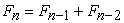for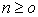,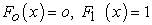(1.1)

Binet Formula for Fibonacci number is defined by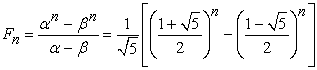(1.2)

In 1883, catalan, E  was considered related set of polynomials which satisfies the recurrence relations.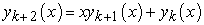The name Fibonacci polynomials is also given to the solution of the relation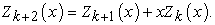(1.3)

With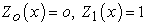, investigated by Jacasthal, E . Byrd, p.F  defined the Fibonacci polynomialsby the recurrence relation.(1.4)

with initial conditions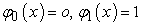swamy, M.N.S  and Hoggatt, V.E.  almost simultaneously defined the Fibonacci polynomialsby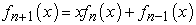(1.5)

with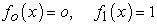.

Here if we put x=1 inwe getwhich if Fibonacci sequence.

Generating function of Fibonacci Polynomials is defined by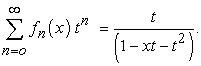Hyper geometric form of generating function of Fibonacci polynomials isTribonacci number Tn  defined by(1.6)

The Tribonacci numbers are (0, 1, 1, 2, 4, 7, 13, 24…).

The tribonacci polynomial tn(x)  satisfies the following recurrence relation: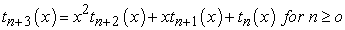and to(x)=o, t1(x)=1 t2(x)=x2.

In this chapter we present some identities of Tribonacci Polynomials by standard methods.

### 2. Tribonacci Polynomials

The Tribonacci polynomial tn(x)  satisfies the following recurrence relation:and to(x)=o, t1(x)=1, t2(x)=x2

The first few Tribonacci polynomials are as follows.

t1(x) = 1

t2(x) = x2

t3(x) = x4 +x

t4(x) = x6 +2x3+1

t5(x) = x8 +3x5+3x2

t6(x) = x10 +4x7+6x4 +2x

t7(x) = x12 +5x9+10x4 +7x3+1

t8(x) = x14 +6x11+15x8 +16x5+6x2…and so on.

Obviously, tn (1) is Just the classical Tribonacci number Tn several basic properties its simple form the Tribonacci polynomials have many interesting properties.

The Generating function of Tribonacci polynomials is defined by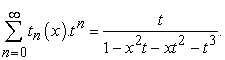(2.1)

Now we will define the Hypergeometric form of generating function of Tribonacci polynomials. In this chapter we shall use following results.

(1)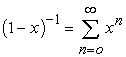(2)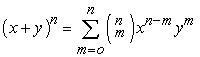(3)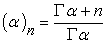(4)### 3. Some Identities of Tribonacci Polynomials

Now we state and prove some Identities of Tribonacci polynomials theorem.

Theorem (3.1).Proof. we know that the generating function of Tribonacci polynomials is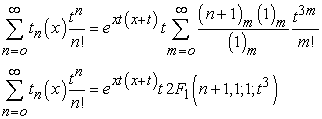Theorem (3.2). recurrence relations by the generating function of Tribonacci polynomials we can easily get following recurrence relations.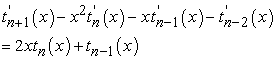and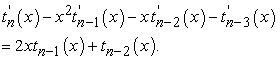Proof. The generating function of Tribonacci polynomials is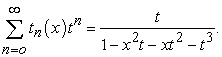Differentiating (2.1) w.r. to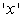we get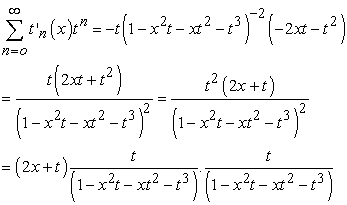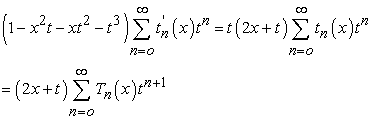Equating the coefficient of tn+1and tn, we getand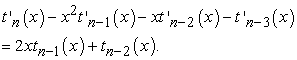Theorem (3.3). Prove that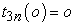and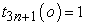.

Proof.

The Generating function of Tribonacci PolynomialsPut x=0 in eqn (2.1) we get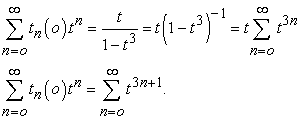Equating the coefficients of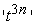and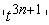on both sides respectively, we getTheorem (3.4).Prove thatProof . Differentiating equation (2.1) w.r.t ‘x', we get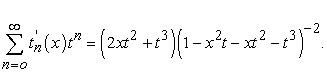Put x=o, we get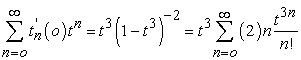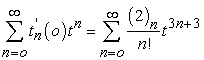on both side respectively, we get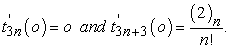### 4. Conclusion

In this paper, we introduce some identities Tribonacci polynomials by standard techniques. Some basic identities are obtained by method of generating function. Also some identities are obtained in hyper geometric form.

### Acknowledgement

The authors would like to thanks the anonymous referee for carefully reading the paper and for their comments.

### References

  Biknell, M Hoggatt, V.E. Jr. Generalized Fibonacci, Polynomials Fibonacci Q.016, 300-303 (1978).In article  Burrage, K generalized Fibonacci polynomials and the functional iteration of Rational Function of degree one Fibonacci Q.28, 175-180 (1990).In article  Byrd, P.F. Expansion of Analytic Functions In polynomials associated with Fibenacci Numbers, the Fibonacci, quarterly, 1(1), (1963), 16-29.In article  Catalon, E., Notes surla Theoric des Fractions continuous sur certain series, mem. Acad. R Belgique, 45, (1883) 1-82.In article  Dilcher, K.A generalization of Fibonacci polynomials of integer order Fibonacci Q.16, 300-303 (1978).In article  Djordjevi´c, G. B. and Srivastava, H. M., Some generalizations of certain sequences associated with the Fibonacci numbers, J. Indonesian Math. Soc. 12 (2006) 99-112.In article  Djordjevi´c, G. B. and Srivastava, H. M., Some generalizations of the incomplete Fibonacci and the incomplete Lucas polynomials, Adv. Stud. Contemp. Math. 11 (2005) 11-32.In article  He, m.x, Ricci, P.E. Asymptotic distribution of zero of weighted fibonacci polynomials, complex var. 28, 375-384 (1996).In article  Hoggatt, V. E., Jr.: Bicknell, Marjporie (1973), “Roots of Fibonacci, Macmillan, New York (1960).In article  Jacosthal, E, Fibonacci Polynomial and kreisteil ungsgleichugen sitzugaberichteder Berlinear, math gesells chaft, 17 (1919), 43-57.In article  Koshy, T. Fibonacci Lucas numbers with application (willey, New York, (2001).In article  Lucy, Joan slater “Generalized Hypergeometric functions” Cambridge University press (1966).In article  Patel. J.M. Advanced problems and solutions, the Fibonacci quarterly, 44(1) (2006) 91.In article  Srivastava, H. M. and. Manocha, H. L, A Treatise on Generating Functions, Halsted Press (Ellis Horwood Limited, Chichester), John Wiley and Sons, New York, Chichester, Brisbane and Toronto, 1984.In article  Swami, M.N.S. problem B-74, The fibonacci Quarterly, 3, (1965), 236. Solution by David Zeitlin, the Fibonacci quarterly, 4(1) (1966), 94.In article  Vorobyou, N.N. The Fibonacci numbers D.C. Health Company, Boston (1963).In article  W.Goh, M.x. He, P.E. Ricci, on the universal zero attrattor of the Tribonacci- Related Polynomials, (2009).In article  Yogesh Kumar Gupta, V. H. Badshah, Mamta Singh, and Kiran Sisodiya, Generalized Additive Coupled Fibonacci Sequences of Third order and Some Identities, International Journal of Innovative Science, Engineering & Technology, Vol. 2 Issue 3, March 2015, 80-85.In article  Yogesh Kumar Gupta, Kiran Sisodiya, Mamta Singh, and Generalization of Fibonacci Sequence and Related Properties, “Research Journal of Computation and Mathematics, Vol. 3, No. 2, (2015), 12-18.In article  Yogesh Kumar Gupta, V. H. Badshah, Mamta Singh, and Kiran Sisodiya, “Diagonal Function of k-Lucas Polynomials.” Turkish Journal of Analysis and Number Theory, vol. 3, no. 2 (2015): 49-52.In article View Article